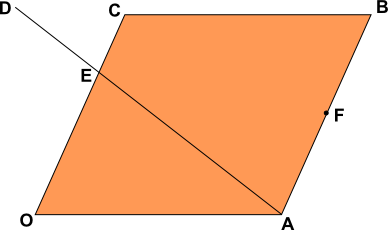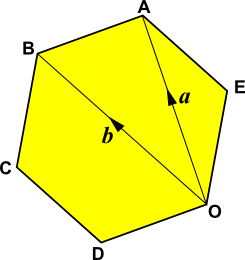# Exam-Style Questions on Vectors

## Problems on Vectors adapted from questions set in previous GCSE Extended exams.

### 1.

IGCSE Extended

OABC is a parallelogram with O as origin. The position vector of A is $$a$$ and the position vector of C is $$c$$.F is the mid-point of AB and the point E divides the line OC such that OE:EC = 2:1.

The point E also divides the line AD such that AE:ED = 3:2.

Find the following in terms of $$a$$ and $$c$$.

(a) $$\overrightarrow{OB}$$

(b) $$\overrightarrow{AC}$$

(c) $$\overrightarrow{AE}$$

(d) the position vector of F.

(e) $$\overrightarrow{AD}$$

(f) $$\overrightarrow{BD}$$

### 2.

IGCSE ExtendedABCDOE is a regular hexagon with O as origin. The position vector of A is $$a$$ and the position vector of B is $$b$$.

Find the following in terms of $$a$$ and $$b$$.

(a) $$\overrightarrow{BA}$$

(b) $$\overrightarrow{OE}$$

(c) the position vector of C.

If the sides of the hexagon are each of length 10cm calculate:

(d) the size of angle $$BCD$$.

(e) the area of triangle $$BCD$$.

(f) the length of the line from B to D.

(g) the area of the hexagon.

### 3.

IGCSE Extended

(a) If A is the point (3,5) write down the position vector of A.

(b) If B is the point (6,9) find $$\mid\overrightarrow{AB} \mid$$ the magnitude of $$\overrightarrow{AB}$$.

The following diagram is not to scale.$$O$$ is the origin, $$\overrightarrow{OP}=p$$ and = $$\overrightarrow{OQ}=q$$.

$$OP$$ is extended to $$R$$ so that $$OP=PR$$.

$$OQ$$ is extended to $$S$$ so that $$OQ=QS$$.

(c) Write down $$\overrightarrow{RQ}$$ in terms of $$p$$ and $$q$$.

(d) $$PS$$ and $$RQ$$ intersect at $$M$$ and $$RM=2 MQ$$.

Use vectors to find the ratio $$PM:PS$$, showing all your working.

The exam-style questions appearing on this site are based on those set in previous examinations (or sample assessment papers for future examinations) by the major examination boards. The wording, diagrams and figures used in these questions have been changed from the originals so that students can have fresh, relevant problem solving practice even if they have previously worked through the related exam paper.

The solutions to the questions on this website are only available to those who have a Transum Subscription.

Exam-Style Questions Main Page

Search for exam-style questions containing a particular word or phrase:

To search the entire Transum website use the search box in the grey area below.